Latest Banking jobs   »   IBPS quant

# Quantitative Aptitude Quiz For IBPS Clerk Mains 2023-16th-September

Q1. The compound interest on a certain sum of money at a certain rate per annum for two years is Rs. 6912 and the simple interest on the same amount of money at the same rate for 3 years is Rs. 2880. Find the principal amount.
(a) Rs 6000
(b) Rs 5400
(c) Rs 4800
(d) Rs 4500
(e) Rs 6300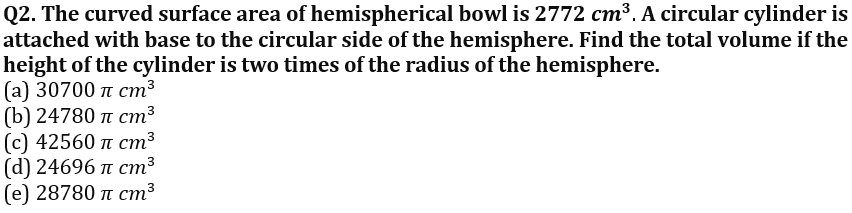Q3. Dharmender sold his bicycle to Shivam at loss of 25%. Shivam repair the bicycle in Rs 250 and then sold to Anurag at profit of 20%. Anurag sold it to Harish at 20% loss. Now, Dharmender bought the bicycle from Harish in Rs 1050. Find the profit/loss of the Dharmender if Harish gain a profit of Rs 90 after selling the bicycle to Dharmender.
(a) Rs 80
(b) Rs 50
(c) Rs 100
(d) Rs 70
(e) Rs 90

Q4. A and B starts a business together. A invests Rs 6300 while B invests 25% more than A. After 8 months, B withdraws 40% of his investment. If at the end of the year, they divide the 75% of the total profit in investment x time ratio and remaining profit in the ratio of 13 : 12, then find the total profit. Profit share of B is Rs 6120.
(a) Rs 12000
(b) Rs 15000
(c) Rs 13000
(d) Rs 18000
(e) Rs 20000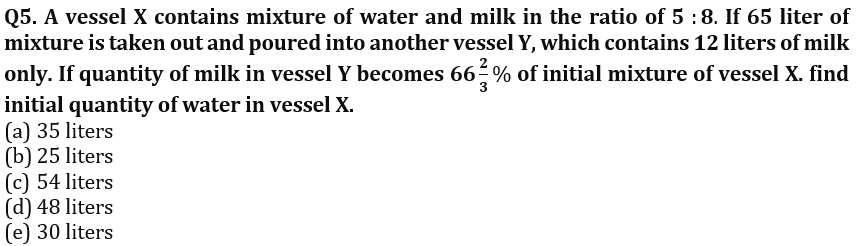Q6.There is two vessels A and B. Vessel A & B contains the mixture of milk, mango juice and water in the ratio of 8 : 5 : 3 & 6 : 5 : 2 respectively. If both vessels are mixed respectively in the ratio of 16 : 13 into another vessel C then the total quantities of mango juice in vessel C will be 20 liters. Find the difference between the quantity of milk and water in vessel C?
(a)15 liters
(b)10 liters
(c)18 liters
(d)25 liters
(e)20 liters

Q7. Satish saved 55% of his monthly income, which he further invested in two different schemes A & B in the ratio of 7 : 5 on CI compounded annually at the rate of 10% p.a. and 20% p.a. respectively. If after two years, Satish gets total interest of Rs. 8074 from both the schemes, then find the total annual saving of Satish?
(a)316,600 Rs.
(b)316,400 Rs.
(c)316,000 Rs.
(d)316,200 Rs.
(e)316,800 Rs.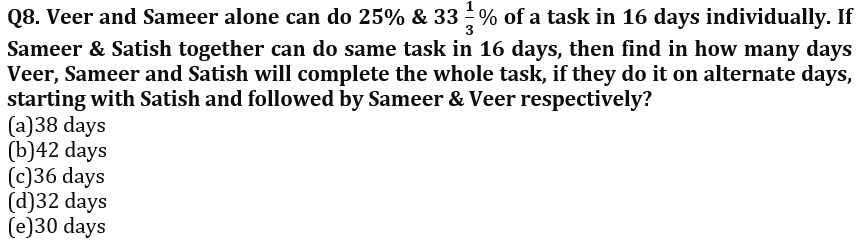Q9. Ratio between length of two trains A & B is 3 : 4 and both the trains are running at the speed of 81 km/hr and 108 km/hr respectively. If both the trains are running in opposite direction, they crossed each other in 8 sec, then find in what time both trains will cross each other when running in same direction?

(a)48 sec

(b)56 sec

(c)44 sec

(d)42 sec

(e)40 sec

Q10. Radius of a cylinder is half of the side of square, whose perimeter is 6 cm more than perimeter of rectangle. If ratio between length to breadth of rectangle is 7 : 2 and between length of rectangle to side of square is 3 : 2. Find volume of cylinder, given height of cylinder is equal to breadth of rectangle?

(a)24,938 cm3
(b)24,948 cm3
(c)24,848 cm3
(d)24,996 cm3
(e)24,918 cm3

Solutions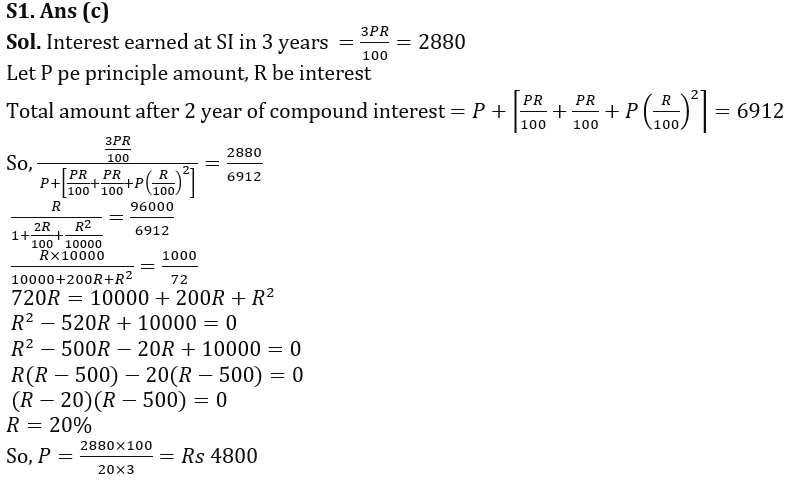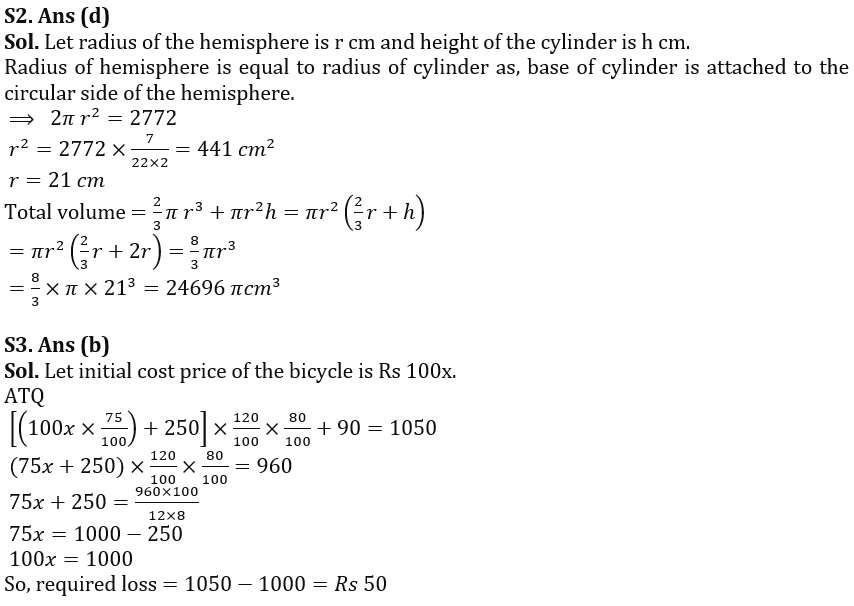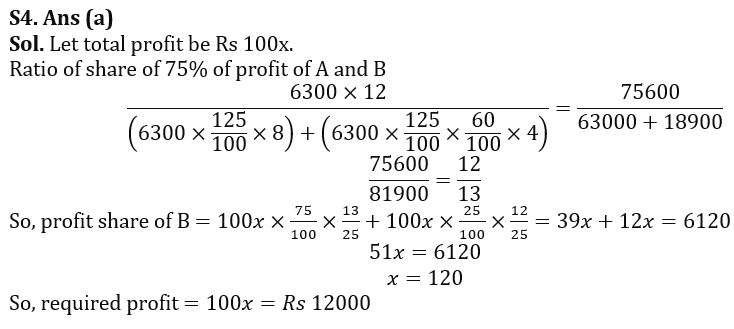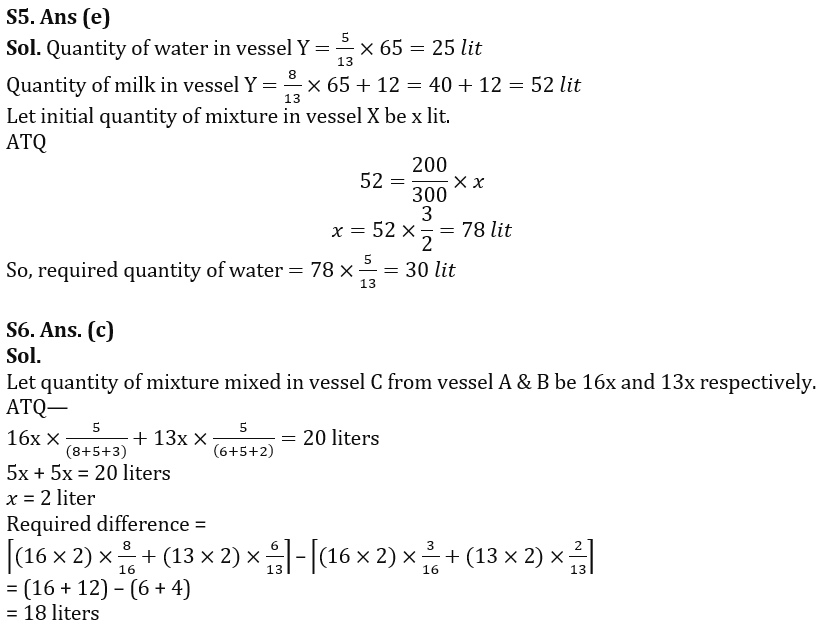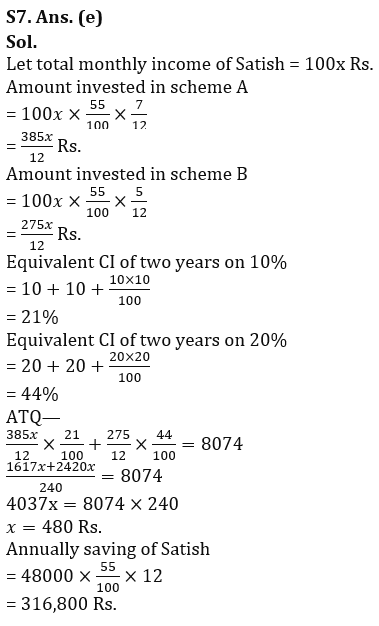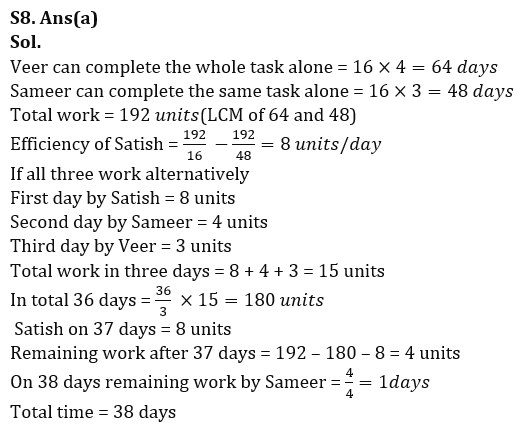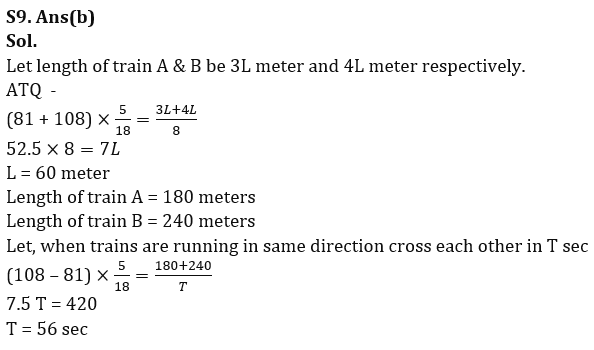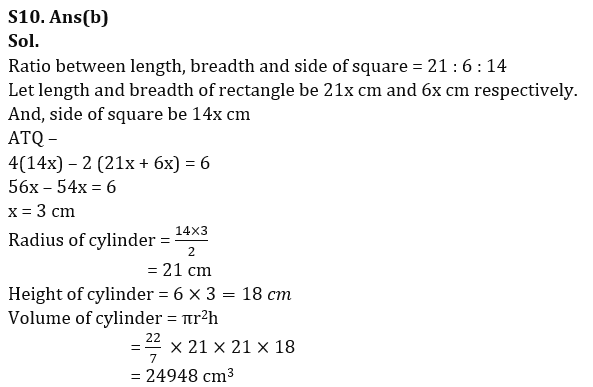## FAQs

### When is the IBPS Clerk Mains exam scheduled for?

The IBPS Clerk Mains exam is scheduled for 7 October 2023.

#### Congratulations!Union Budget 2023-24: Free PDF